Question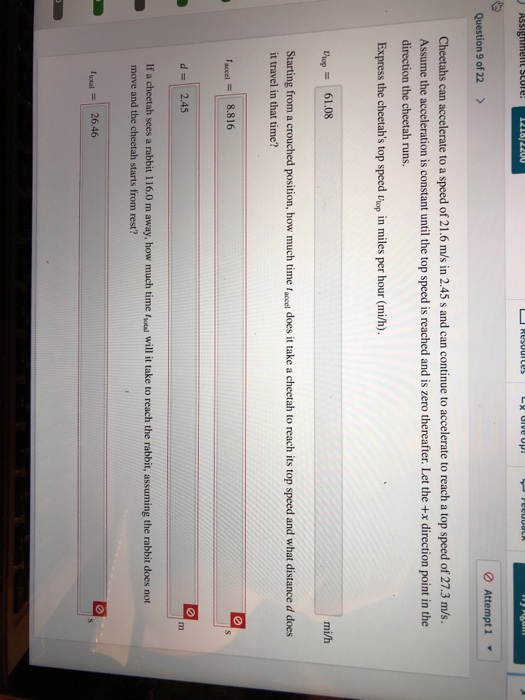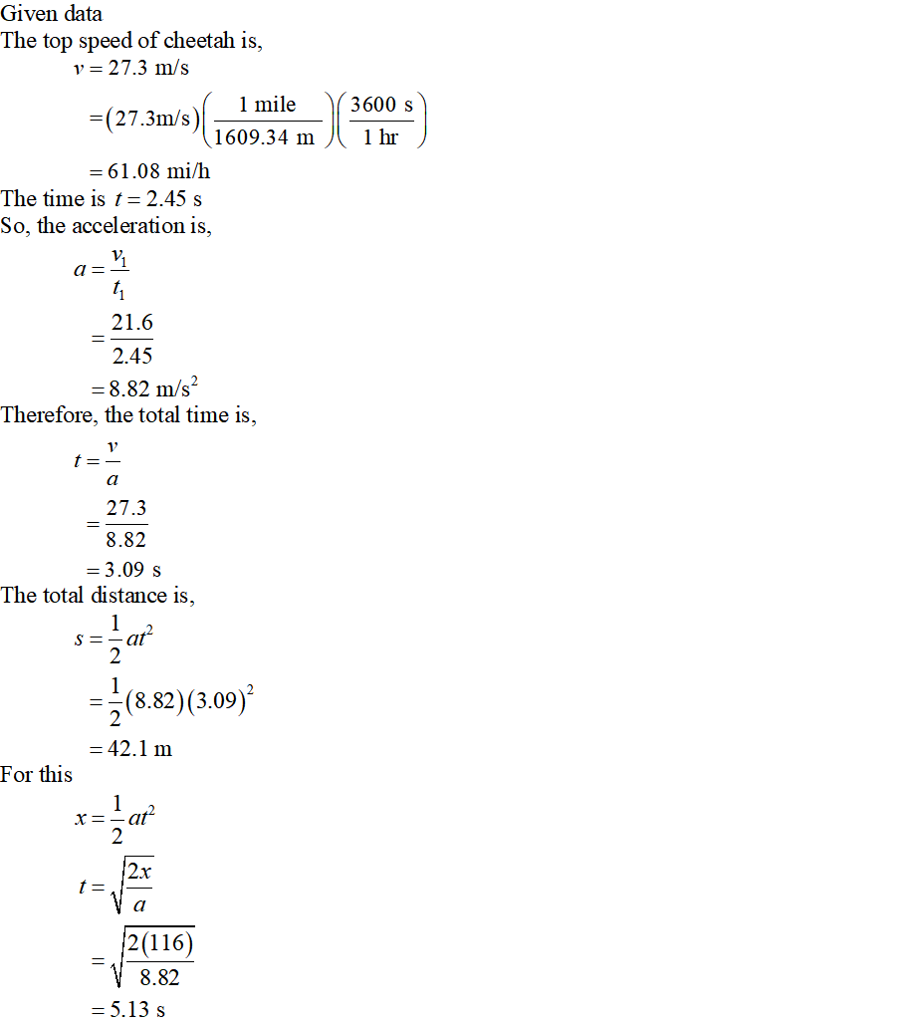#### Earn Coins

Coins can be redeemed for fabulous gifts.

Similar Homework Help Questions
• ### Cheetahs can accelerate to a speed of 21.4 m/s in 2.55 s and can continue to...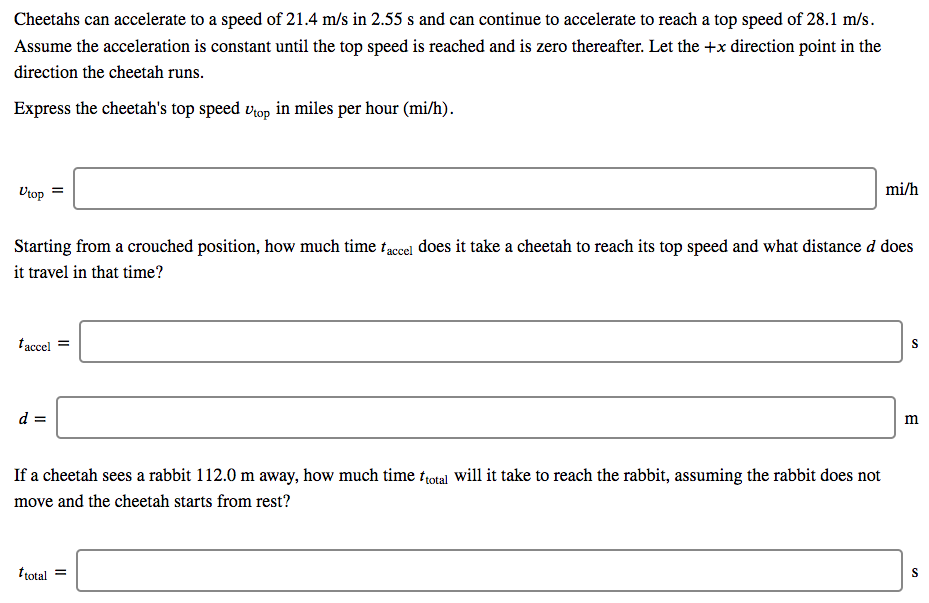Cheetahs can accelerate to a speed of 21.4 m/s in 2.55 s and can continue to accelerate to reach a top speed of 28.1 m/s. Assume the acceleration is constant until the top speed is reached and is zero thereafter. Let the +x direction point in the direction the cheetah runs. Express the cheetah's top speed vop in miles per hour (mi/h). uop- mi/h Starting from a crouched position, how much time faccel does it take a cheetah to reach...

• ### Cheetahs can accelerate to a speed of 19.8 m/s in 2.65 s and can continue to...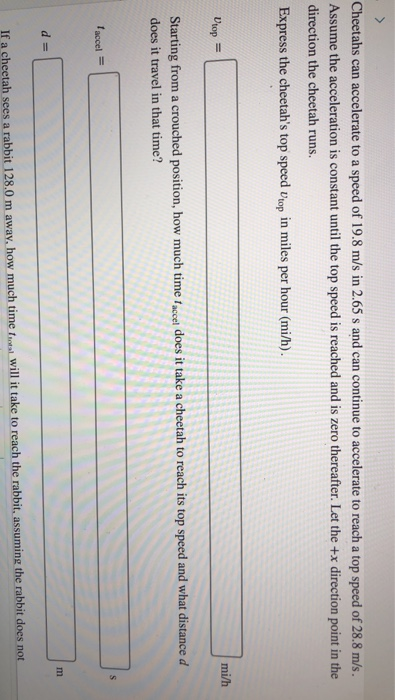Cheetahs can accelerate to a speed of 19.8 m/s in 2.65 s and can continue to accelerate to reach a top speed of 28.8 m/s. Assume the acceleration is constant until the top speed is reached and is zero thereafter. Let the +x direction point in the direction the cheetah runs. Express the cheetah's top speed Vop in miles per hour (mi/h) mi/h Starting from a crouched position, how much time tae does it take a cheetah to reach its...

• ### Cheetahs can accelerate to a speed of 21.6 m/s in 2.65 s and can continue to...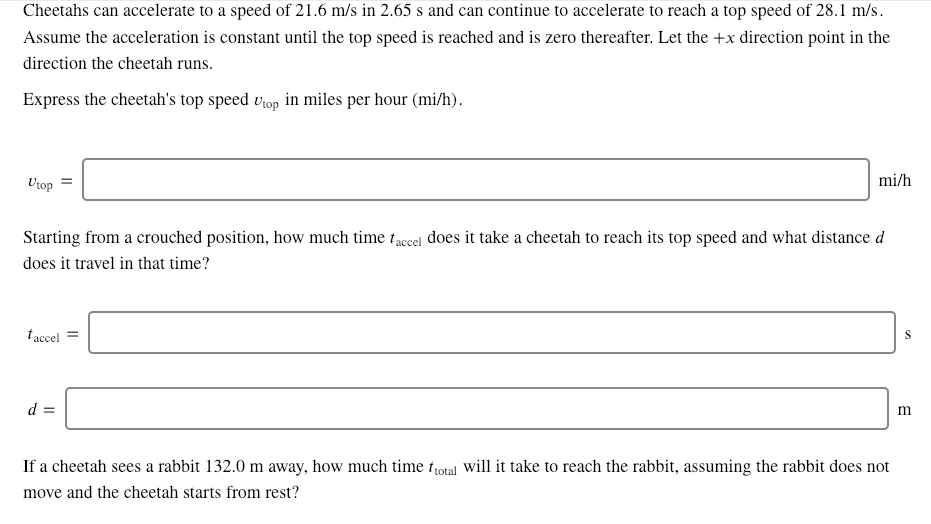Cheetahs can accelerate to a speed of 21.6 m/s in 2.65 s and can continue to accelerate to reach a top speed of 28.1 m/s Assume the acceleration is constant until the top speed is reached and is zero thereafter. Let the +x direction point in the direction the cheetah runs. Express the cheetah's top speed op in miles per hour (mi/h) mi/h top Starting from a crouched position, how much time taccel does it take a cheetah to reach...

• ### Cheetahs can accelerate to a speed of 19.5 m/s in 2.45 s and can continue to...

Cheetahs can accelerate to a speed of 19.5 m/s in 2.45 s and can continue to accelerate to reach a top speed of 29.5 m/s . Assume the acceleration is constant until the top speed is reached and is zero thereafter. Let the +? direction point in the direction the cheetah runs. Express the cheetah's top speed ?top in miles per hour (mi/h) . ?top= mi/h Starting from a crouched position, how much time ?accel does it take a cheetah...

• ### Resources Cheetahs can accelerate to a speed of 21.9 m/s in 2.60 s and can continue...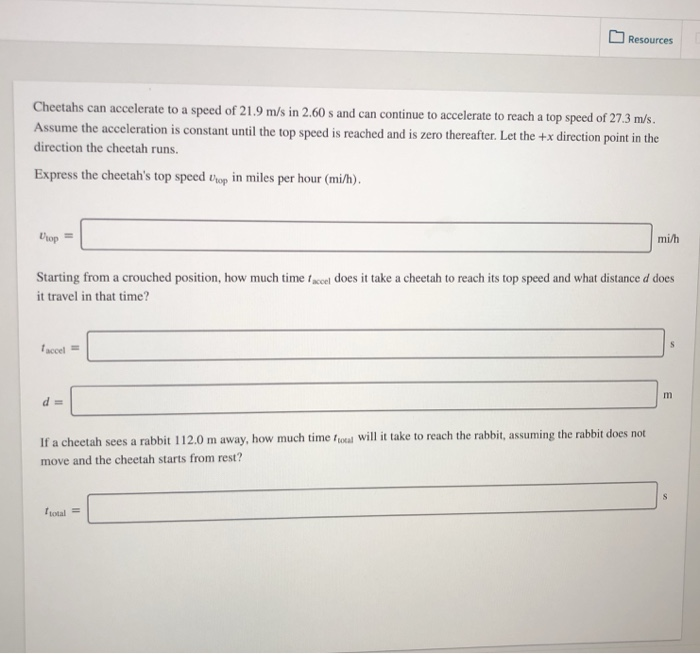Resources Cheetahs can accelerate to a speed of 21.9 m/s in 2.60 s and can continue to accelerate to reach a top speed of 27 3 m/ Assume the acceleration is constant until the top speed is reached and is zero thereafter. Let the +x direction point in the direction the cheetah runs. Express the cheetah's top speed trop in miles per hour (mi/h). mi/h Starting from a crouched position, how much time faccel does it take a cheetah to...

• ### Cheetahs can accelerate to a speed of 19.3 m/s in 2.70 s and can continue to...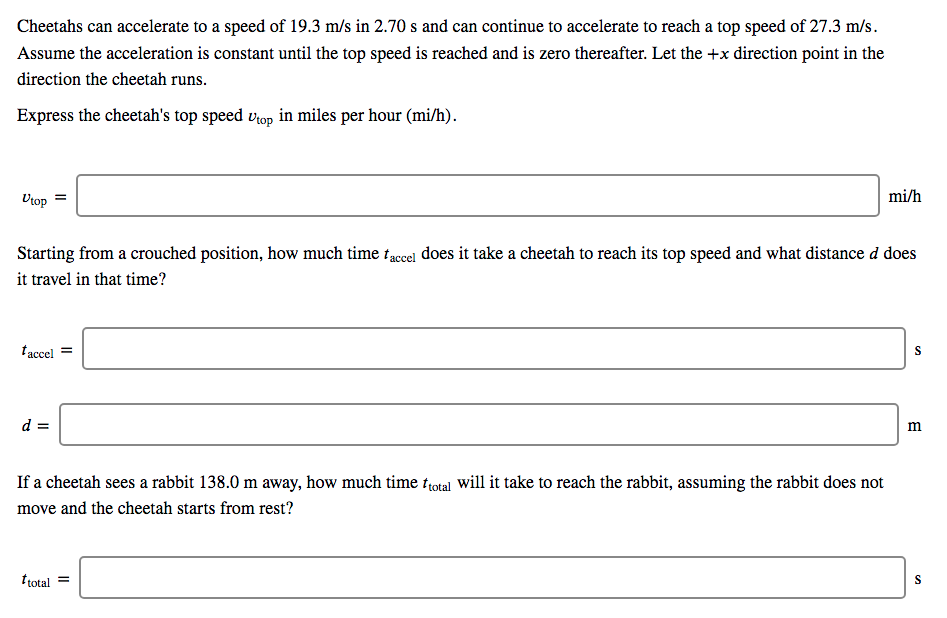Cheetahs can accelerate to a speed of 19.3 m/s in 2.70 s and can continue to accelerate to reach a top speed of 27.3 m/s. Assume the acceleration is constant until the top speed is reached and is zero thereafter. Let the +x direction point in the direction the cheetah runs Express the cheetah's top speed vop in miles per hour (mi/h). mi/h Starting from a crouched position, how much time faccel does it take a cheetah to reach its...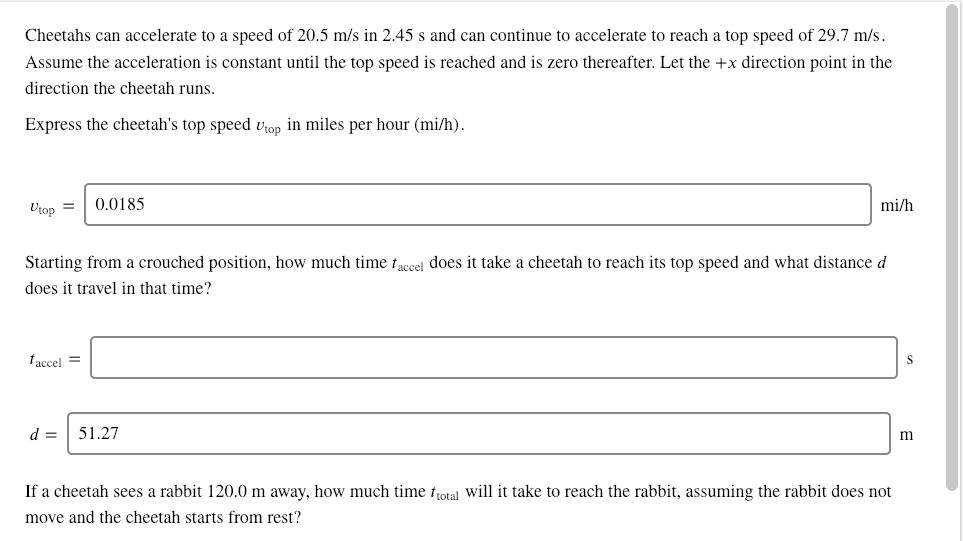Please help! a bit confused. bottom just asks for total Cheetahs can accelerate to a speed of 20.5 m/s in 2.45 s and can continue to accelerate to reach a top speed of 29.7 m/s. Assume the acceleration is constant until the top speed is reached and is zero thereafter. Let the x direction point in the direction the cheetah runs Express the cheetah's top speed vhop in miles per hour (mi/h). mi/h top0.0185 Starting from a crouched position, how...

• ### Cheetahs can accelerate to a speed of 20.4 m/s in 2.50 s and can continue to...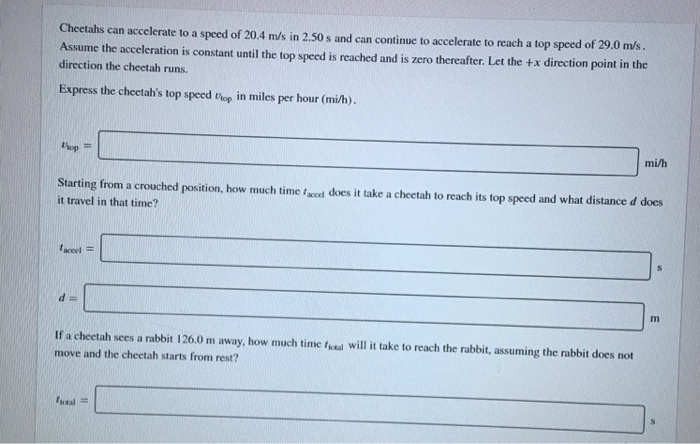Cheetahs can accelerate to a speed of 20.4 m/s in 2.50 s and can continue to accelerate to reach a top speed of 29.0 m/s. direction the cheetah runs. Express the cheetah's top speed brop in miles per hour (mi/h). Assume the acceleration is constant until the top speed is reached and is zero thereafter. Let the +x direction point in tho mi/h Starting from a crouched position, how much time facci does it take a cheetah to reach its...

• ### Cheetahs can accelerate to a speed of 21.3 m/s in 2.70 s and can continue to...

Cheetahs can accelerate to a speed of 21.3 m/s in 2.70 s and can continue to accelerate to reach a top speed of 29.9 m/s. Assume the acceleration is constant until the top speed is reached and is zero thereafter. Let the +? direction point in the direction the cheetah runs. Express the cheetah's top speed ?topvtop in miles per hour (mi/h). Starting from a crouched position, how much time ?acceltaccel does it take a cheetah to reach its top...

• ### 1. Cheetahs can accelerate to a speed of 20.0 m/s in 2.50 s and can continue...

1. Cheetahs can accelerate to a speed of 20.0 m/s in 2.50 s and can continue to accelerate to reach a top speed of 27.6 m/s. Assume the acceleration is constant until the top speed is reached and is zero thereafter. Let the +?+x direction point in the direction the cheetah runs. Express the cheetah's top speed ?top in miles per hour (mi/h)(mi/h) . vtop = Starting from a crouched position, how much time ?accel does it take a cheetah...